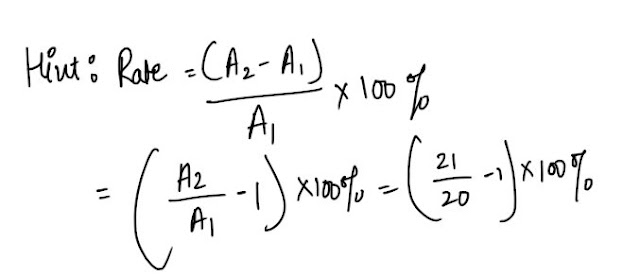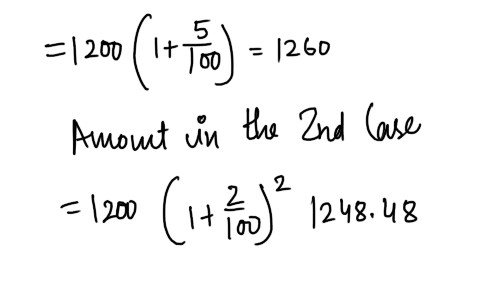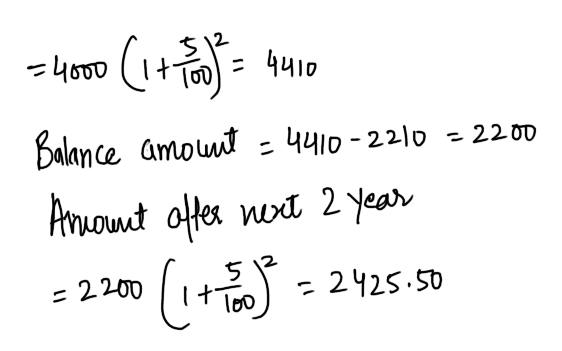# Compound Interest : 10 Repeated Questions for SBI Clerk Mains#### Ques 1.

If the amounts for a fixed principal after 3 years and 2 years at a certain rate of compound interest are in the ratio of 21 : 20 , then the rate of interest ie
(a) 7%
(b) 6%
(c) 5%
(d) 4%
Ans 1.  5 %#### Ques 2.

The difference between compound interest and simple interest on a certain sum of money at 15% per annum in 2 years is Rs 225. Find the sum .
(a) Rs 12000
(b) Rs 11000
(c) Rs 10000
(d) Rs 15000
Ans 2.  Rs 10000#### Ques 3.

A money lender lends out a part of Rs 3364 at 5% compound interest for 3 years and the rest also at 5% compound interest , but for 5 years. If the final amount in both the cases is same , what is the amount lent out for 5 years ?
(a) Rs 2000
(b) Rs 1760
(c) Rs 1600
(d) Rs 1000
Ans 3.  Rs 1600#### Ques 4.

The population of a town increased by 5% of what it had been at the beginning of each year. If the population was 441000 in 1997, find the population in 1995.
(a) 430000
(b) 410000
(c) 400000
(d) 406000
Ans 4.  400000#### Ques 5.

Calculate the compound interest on Rs 1875 for 3 years if the rates of interest per annum are 5%, 6 2/3% and 8 1/3% respectively.
(a) Rs 400
(b) Rs 560
(c) Rs 520
(d) Rs 350
Ans 5.  Rs 400#### Ques 6.

Mr. Sundaram takes a loan of Rs 1200 to be paid after 1 year . He is to pay interest either at 5% p.a. compounded annually or at 4% p.a. compounded six monthly .which option in better for him and by how much ?
(a) IInd option, Rs 11.50
(b) IInd option, Rs 12.75
(c) IInd option , Rs 13.50
(d) 1st option , Rs 10
Ans 6. IInd  option, Rs 11.50#### Ques 7.

Rajan borrowed Rs 4000 at 5% p.a. compound interest. After 2 years ,he repaid Rs 2210 and after 2 more years, the balance witth interest . what was the total amount that he paid as interest ?
(a) Rs 635.50
(b) Rs 613.50
(c) Rs 675.50
(d) Rs 653.50
Ans 7.  Rs 635.50#### Ques 8.

Indu was Bindu Rs 1250 on compound interest fro 2 years at 4% per annum. How much loss would indu have suffered had she given it to Bindu for 2 years at 4 % per annum simple interest ?
(a) Rs 10
(b) Rs 2
(c) Rs 5
(d) None of these
Ans 8.  Rs 2